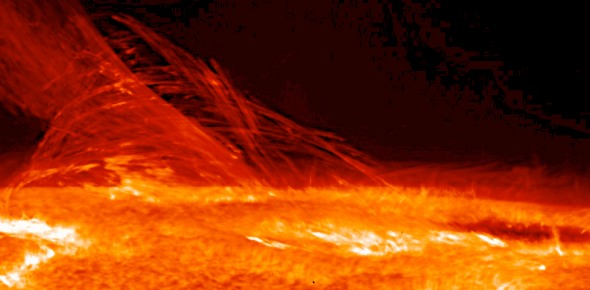# Heat, Light And Sound Test

7 Questions | Total Attempts: 3502SettingsJust do it!

• 1.
Energy is the ability to do:
• A.

Work

• B.

Play

• C.

Study

• D.

Teach

• 2.
The two main types of energy are:
• A.

Heat and light

• B.

Kinetic and Potential

• C.

• D.

Kinetic and Heat

• 3.
What is energy measured with?
• A.

Joules

• B.

Litres

• C.

Kilograms

• D.

Decibels

• 4.
What is the source of energy?
• A.

The Sun

• B.

Earth

• C.

The Moon

• D.

The stars

• 5.
Heat energy can:
• A.

Conduct electricity

• B.

Set something on fire

• C.

Increase the temperature of a substance

• D.

Freeze things

• 6.
What happens when an object is hot?
• A.

The particles disappear

• B.

The particles vibrate

• C.

The particles stay in one spot

• D.

The particles vibrate more rapidly

• 7.
What are three ways that heat can move?
• A.

• B.Back to top